# 常见几种java排序算法

### 1.插入排序

将待插元素，依次与已排序好的子数列元素从后到前进行比较，如果当前元素值比待插元素值大，则将移位到与其相邻的后一个位置，否则直接将待插元素插入当前元素相邻的后一位置，因为说明已经找到插入点的最终位置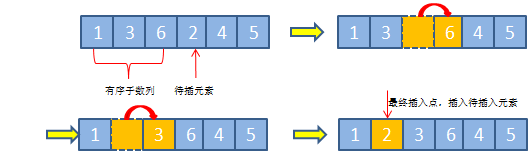public class InsertSort {

public static void sort(int[] arr) {
if (arr.length >= 2) {
for (int i = 1; i < arr.length; i++) {
//挖出一个要用来插入的值,同时位置上留下一个可以存新的值的坑
int x = arr[i];
int j = i - 1;
//在前面有一个或连续多个值比x大的时候,一直循环往前面找,将x插入到这串值前面
while (j >= 0 && arr[j] > x) {
//当arr[j]比x大的时候,将j向后移一位,正好填到坑中
arr[j + 1] = arr[j];
j--;
}
//将x插入到最前面
arr[j + 1] = x;
}
}
}
}


### 2.分治排序法,快速排序法

1. 选择待排数列的首部第一个元素为基准元素x，设置两指针，分别指向数列首尾部位置，假设两指针分别设为ij
2. 每次遍历的过程是这样的，首先从右到左遍历指针j所指向的元素，直到j指向的元素值小于基准元素x时，停止遍历，将其放到i的位置(因为i的值已经拷贝成了基准x腾出了位置)
3. i往右挪一步, i++,接着轮到指针i左到右遍历,直到i所指向的元素值大于基准元素x时，停止遍历，将其放到j的位置(因为上面一步j的值已经占用到了i的位置,腾出位置了)
4. 依此类推，两边轮流遍历, 直到指针i与指针j相等或者大于(实际肯定是i==j)时，停止外部循环。此时必定左边都是比x小的, 右边是比x大的.
5. 最后直接将基准元素x直接放置于指针i所指向的位置即可
6. 完成分区操作, 从i的位置一分为二, 左边和右边再递归执行上面的操作. 层层细分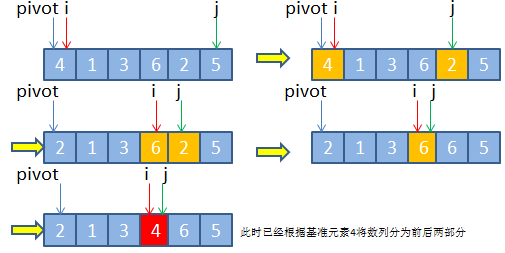public class QuickSort {

public static void sort(int[] arr,int begin,int end) {
//先定义两个参数接收排序起始值和结束值
int a = begin;
int b = end;
//先判断a是否大于b

if (a >= b) {
//没必要排序
return;
}
//基准数,默认设置为第一个值
int x = arr[a];

//循环
while (a < b) {
//从后往前找,找到一个比基准数x小的值,赋给arr[a]
//如果a和b的逻辑正确--a<b ,并且最后一个值arr[b]>x,就一直往下找,直到找到后面的值大于x
while (a < b && arr[b] >= x) {
b--;
}
//跳出循环,两种情况,一是a和b的逻辑不对了,a>=b,这时候排序结束.二是在后面找到了比x小的值
if (a < b) {
//将这时候找到的arr[b]放到最前面arr[a]
arr[a] = arr[b];
//排序的起始位置后移一位
a++;
}

//从前往后找,找到一个比基准数x大的值,放在最后面arr[b]
while (a < b && arr[a] <= x) {
a++;
}
if (a < b) {
arr[b] = arr[a];
//排序的终止位置前移一位
b--;
}
}
//跳出循环 a < b的逻辑不成立了,a==b重合了,此时将x赋值回去arr[a]
arr[a] = x;
//调用递归函数,再细分再排序
sort(arr,begin,a-1);
sort(arr,a+1,end);
}
}


### 3.冒泡排序 low版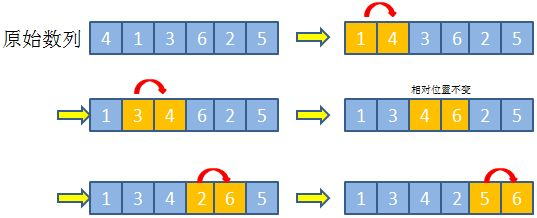public class MaoPao {

public static void  sort(int[] arr){
for (int i = 1; i < arr.length; i++) {  //第一层for循环,用来控制冒泡的次数
for (int j = 0; j < arr.length-1; j++) { //第二层for循环,用来控制冒泡一层层到最后
//如果前一个数比后一个数大,两者调换 ,意味着泡泡向上走了一层
if (arr[j] > arr[j+1] ){
int temp = arr[j];
arr[j] = arr[j+1];
arr[j+1] = temp;
}
}
}
}
}



### 4.冒泡排序 bigger版

1. 第一点是加入了一个布尔值,判断第二层循环中的调换有没有执行,如果没有进行两两调换,说明后面都已经排好序了,已经不需要再循环了,直接跳出循环,排序结束.
2. 第二点是第二层循环不再循环到arr.length - 1,因为外面的i循环递增一次,说明数组最后就多了一个排好序的大泡泡.第二层循环也就不需要到最末尾一位了,可以提前结束循环
 /**
* 终极版冒泡排序
* 加入一个布尔变量,如果内循环没有交换值,说明已经排序完成,提前终止
* @param arr
*/
public static void sortPlus(int[] arr){
if(arr != null && arr.length > 1){
for(int i = 0; i < arr.length - 1; i++){
// 初始化一个布尔值
boolean flag = true;
for(int j = 0; j < arr.length - i - 1 ; j++){
if(arr[j] > arr[j+1]){
// 调换
int temp;
temp = arr[j];
arr[j] = arr[j+1];
arr[j+1] = temp;
// 改变flag
flag = false;
}
}
if(flag){
break;
}
}
}
}



### 5.选择排序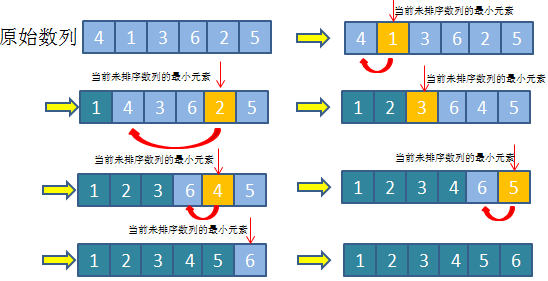public static void sort(int[] arr){
for(int i = 0; i < arr.length - 1 ; i++){
int min = i; // 遍历的区间最小的值
for (int j = i + 1; j < arr.length ;j++){
if(arr[j] < arr[min]){
// 找到当前遍历区间最小的值的索引
min = j;
}
}
if(min != i){
// 发生了调换
int temp =  arr[min];
arr[min] = arr[i];
arr[i] = temp;
}
}
}


### 6. 归并排序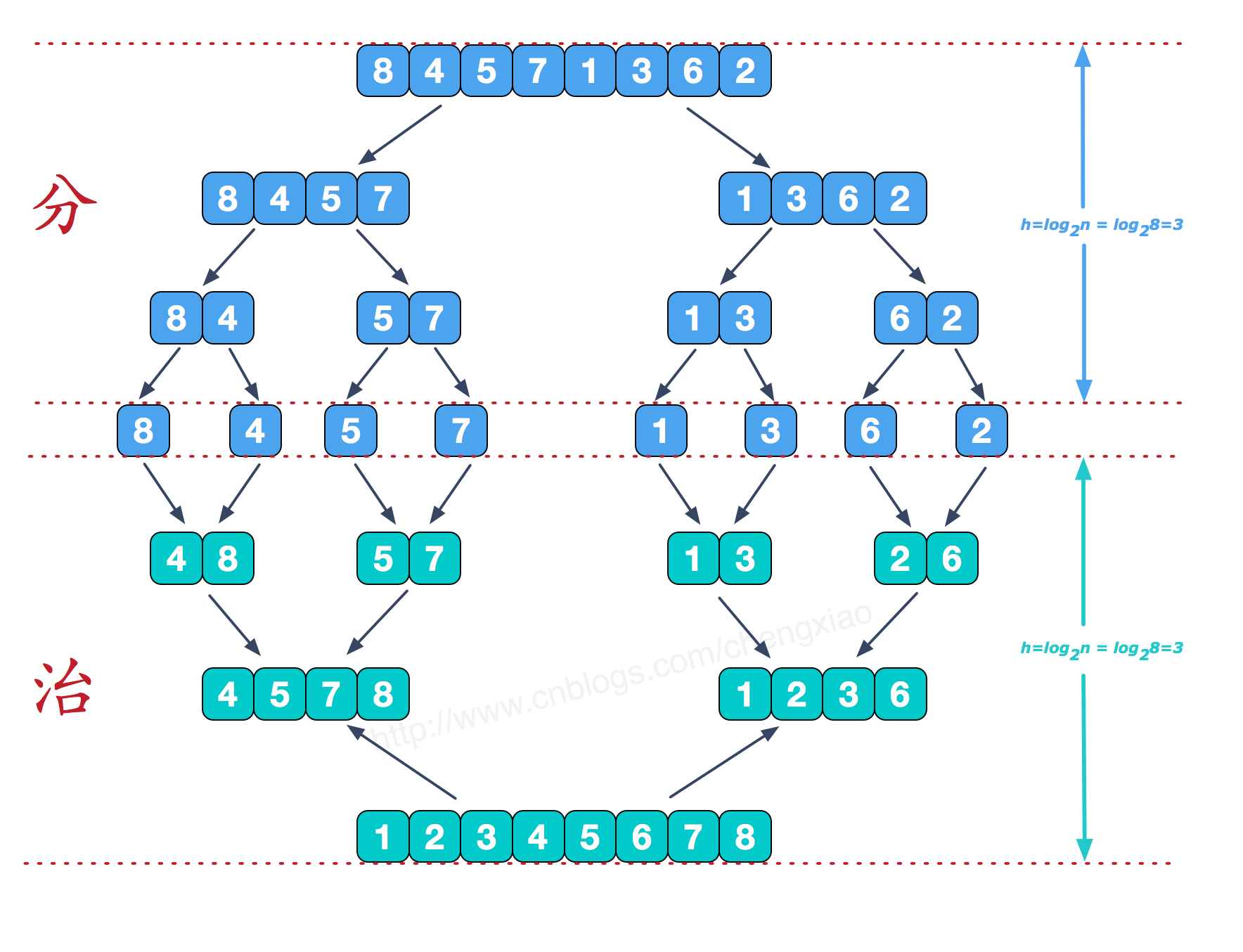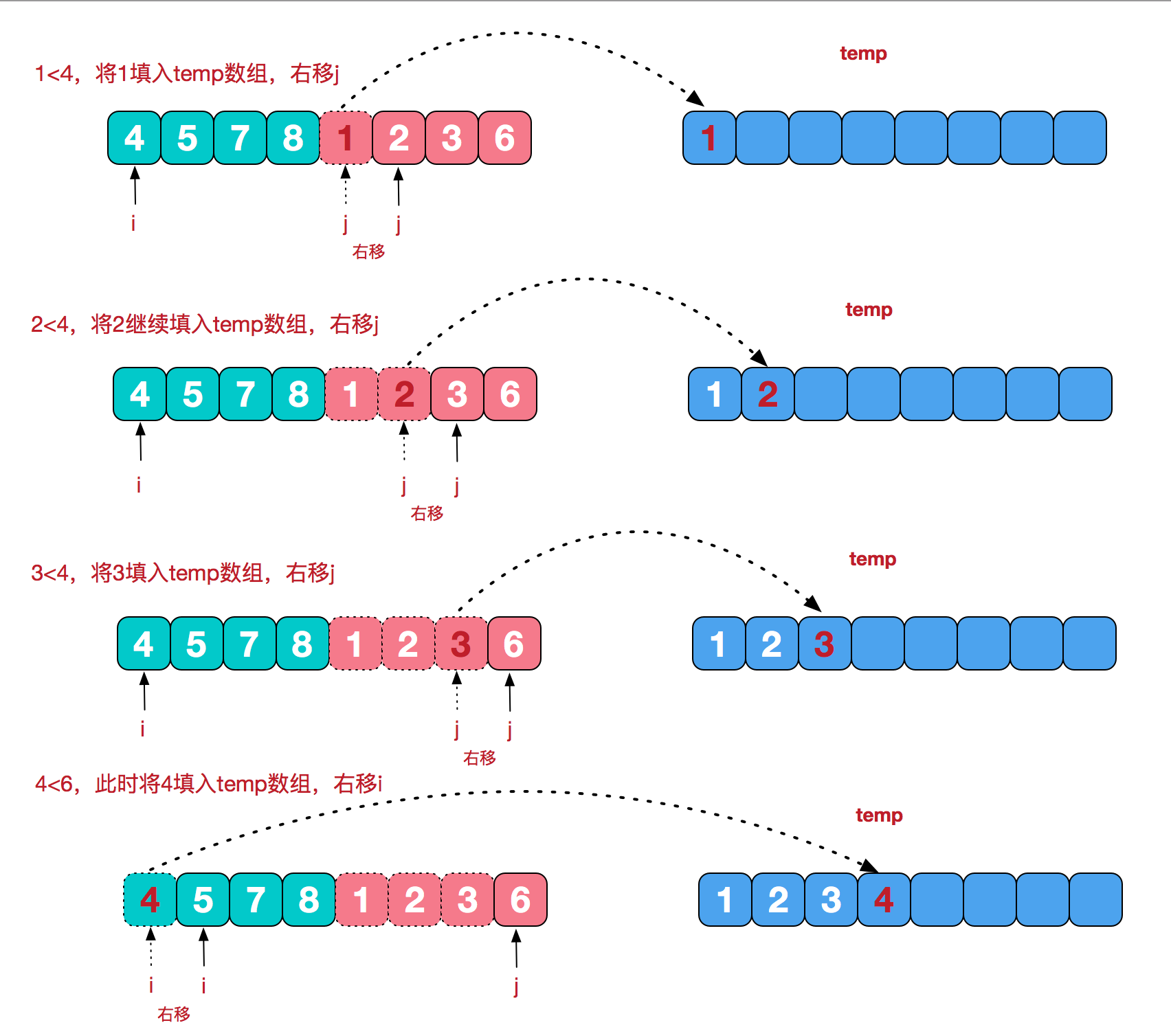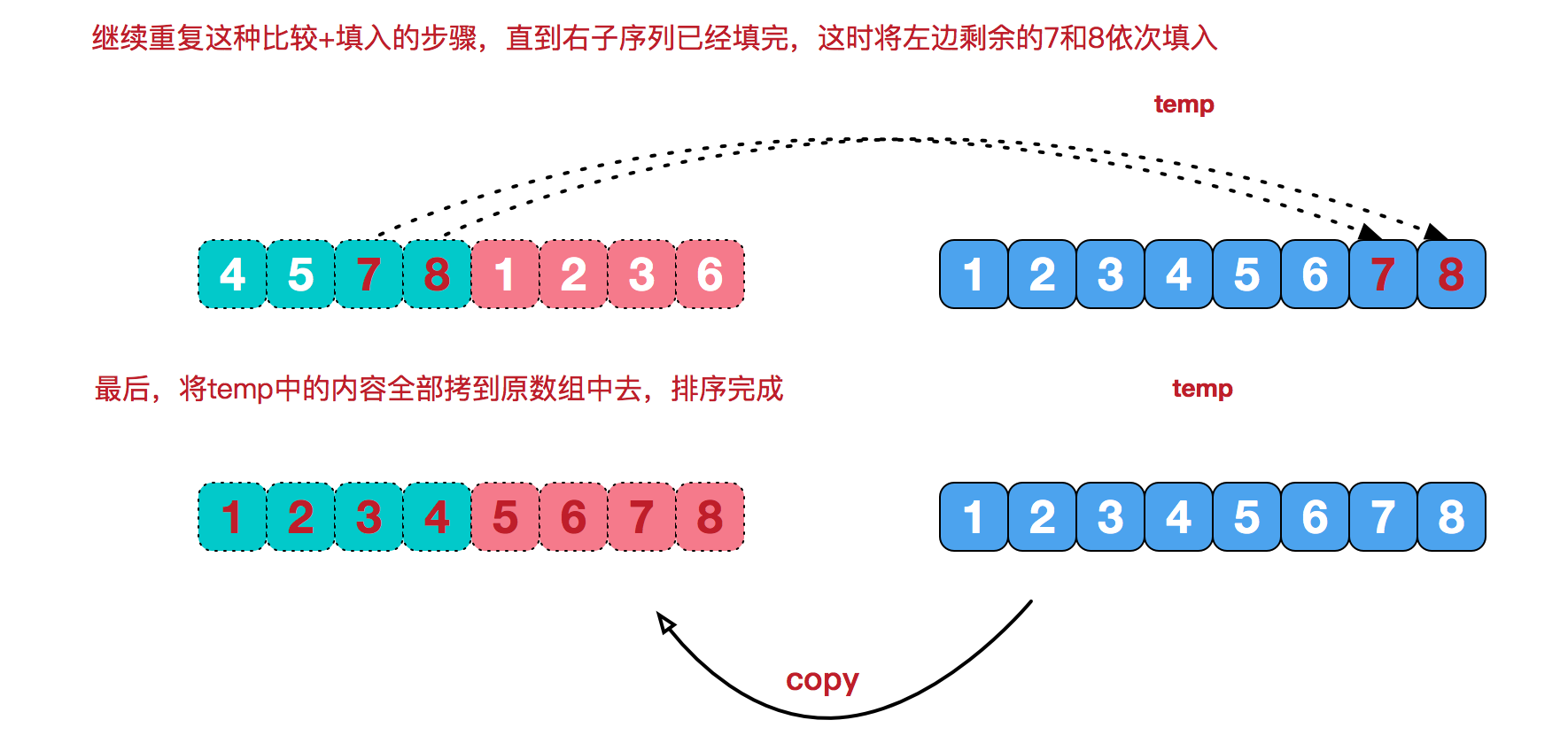public static void mergeSort(int[] a,int s,int e){
int m = (s + e) / 2;
if (s < e){
mergeSort(a,s,m);
mergeSort(a,m+1,e);
//归并
merge(a,s,m,e);
}
}

private static void merge(int[] a, int s, int m, int e) {
//初始化一个从起始s到终止e的一个数组
int[] temp = new int[(e - s) + 1];
//左起始指针
int l = s;
//右起始指针
int r = m+1;
int i = 0;
//将s-e这段数据在逻辑上一分为二,l-m为一个左边的数组,r-e为一个右边的数组,两边都是有序的
//从两边的第一个指针开始遍历,将其中小的那个值放在temp数组中
while (l <= m && r <= e){
if (a[l] < a[r]){
temp[i++] = a[l++];
}else{
temp[i++] = a[r++];
}
}

//将两个数组剩余的数放到temp中
while (l <= m){
temp[i++] = a[l++];
}
while (r <= e){
temp[i++] = a[r++];
}

//将temp数组覆盖原数组
for (int n = 0; n < temp.length; n++) {
a[s+n] = temp[n];
}

}


### 7. 其他排序

private static void arraysSort(int[] a){
Arrays.sort(a);
}


private static void listSort(int[] a){
List<Integer> integers = Ints.asList(a);
Collections.sort(integers);
integers.toArray(new Integer[a.length]);
}


### 7. 比较

public static void main(String[] args) {

int[] arr = new int;
int[] a =getRandomArr(arr);
int[] b = a.clone();
int[] c = b.clone();
int[] d = b.clone();
int[] e = b.clone();
int[] f = b.clone();
int[] g = b.clone();
int[] h = b.clone();

long s = Clock.systemDefaultZone().millis();
quickSort(a, 0, a.length - 1);
System.out.println("quickSort耗时: " + (Clock.systemDefaultZone().millis() - s) + " ms");

s = Clock.systemDefaultZone().millis();
mergeSort(b,0,b.length-1);
System.out.println("mergeSort: " + (Clock.systemDefaultZone().millis() - s) + " ms");

s = Clock.systemDefaultZone().millis();
listSort(c);
System.out.println("listSort耗时: " + (Clock.systemDefaultZone().millis() - s) + " ms");

s = Clock.systemDefaultZone().millis();
arraysSort(d);
System.out.println("arraysSort耗时: " + (Clock.systemDefaultZone().millis() - s) + " ms");

s = Clock.systemDefaultZone().millis();
maoPaoSort(e);
System.out.println("maoPaoSort耗时: " + (Clock.systemDefaultZone().millis() - s) + " ms");

s = Clock.systemDefaultZone().millis();
maoPaoSortPlus(f);
System.out.println("maoPaoSortPlus耗时: " + (Clock.systemDefaultZone().millis() - s) + " ms");

s = Clock.systemDefaultZone().millis();
insertSort(g);
System.out.println("insertSort耗时: " + (Clock.systemDefaultZone().millis() - s) + " ms");

s = Clock.systemDefaultZone().millis();
selectSort(h);
System.out.println("selectSort耗时: " + (Clock.systemDefaultZone().millis() - s) + " ms");

}

/**
* 获取一个打乱的数组
* @param arr
*/
private static int[] getRandomArr(int[] arr) {
for (int i = 0; i < arr.length; i++) {
arr[i] = new Random().nextInt(arr.length);
}
return arr;
}


//---1k---
quickSort耗时: 0 ms
mergeSort: 1 ms
listSort耗时: 7 ms
arraysSort耗时: 1 ms
maoPaoSort耗时: 3 ms
maoPaoSortPlus耗时: 4 ms
insertSort耗时: 2 ms
selectSort耗时: 3 ms
//---1w---
quickSort耗时: 2 ms
mergeSort: 3 ms
listSort耗时: 19 ms
arraysSort耗时: 4 ms
maoPaoSort耗时: 166 ms
maoPaoSortPlus耗时: 122 ms
insertSort耗时: 12 ms
selectSort耗时: 52 ms

//---10w---
quickSort耗时: 14 ms
mergeSort: 19 ms
listSort耗时: 65 ms
arraysSort耗时: 12 ms
maoPaoSort耗时: 15242 ms
maoPaoSortPlus耗时: 15044 ms
insertSort耗时: 797 ms
selectSort耗时: 4073 ms
//---20w---
quickSort耗时: 26 ms
mergeSort: 34 ms
listSort耗时: 102 ms
arraysSort耗时: 60 ms
maoPaoSort耗时: 60811 ms
maoPaoSortPlus耗时: 60378 ms
insertSort耗时: 3279 ms
selectSort耗时: 15762 ms


08-315万+
05-17378904-282万+
07-168万+
10-1465
09-135997
10-23134
04-171万+
09-21
05-317113
11-292万+
06-1015万+
12-171630
08-191038
04-22253
06-073848
06-281万+
04-15
03-056536
07-022947
©️2020 CSDN 皮肤主题: 酷酷鲨 设计师:CSDN官方博客